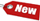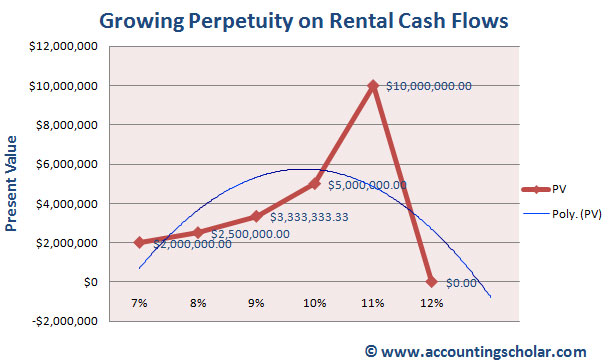Browse Accounting Lessons HereAccounting Terms & DefinitionsAccounting for Merchandising ActivitiesDebits and Credits (Double Entry Accounting)Business Valuation FormulasTime Value of Money & Present/Future ValuesComplex Debt & Equity InstrumentsCommon Stock & Shareholder's EquityAccounting & Finance RatiosValuing Common StockCorporate Income TaxesLower of Cost or Market (LCM) & Inventory ValuationChart of Accounts & BookkeepingBonds Payable & Long Term LiabilitiesCapital AssetsGAAP, Accrual & Cash Accounting, Information Commodity, Internal Controls & MaterialityWhat category of browser are you on this website? Accounting student (homework help) Finance professor (university research) Accounting manager (at work) Other Explore Careers in Accounting and FinanceVisit our section on Careers in Accounting & Finance to explore vast opportunities in this industry.

Chapter 4.18® - Accounting for Growing Perpetuities & Simplified Formula for Present Value of Growing Perpetuity - Example of Growing Perpetuity on Rental Cash Flows

The perpetuities discussed so far are annuities with constant equal payments over their lives. However, there are many perpetuity investments out there that have growing payments. For instance, imagine a landlord receives \$100,000 in cash flows after expenses from an apartment building that he owns. These cash flows are expected to rise at 7% a year level, and we assume that this rise will continue indefinitely. Having assumed a discount rate of 12% a year, this stream of future cash flows is known as a growing perpetuity. The formula for a growing perpetuity is:

 PV = (( C / 1 + r)) + ( (C x (1 + g)) / (1 + r)2) + ( (C x (1 + g)2) / (1 + r)3 .... PV = (( C x (1 + g)N-1) )/ (1 + r)N

Where:

 C = cash flow to be received in one period g = growth rate per period r = interest or discount rate

Not to worry, this formula can be simplified further to arrive at the kind of formula we would enjoy using.

Simplified Formula for Present Value of Growing Perpetuity

 PV = C / (r – g)

Where:

 C = cash flow to be received in one period g = growth rate per period r = interest or discount rate

For instance, imagine a landlord receives \$100,000 in cash flows after expenses from an apartment building that he owns. These cash flows are expected to rise at 7% a year level, and we assume that this rise will continue indefinitely. Having assumed a discount rate of 12% a year, what is the present value of this perpetuity?

 PV = C / (r – g) PV = \$100,000 / (0.12 – 0.07) PV = \$100,000 / 0.05 PV = \$2,000,000

Here are 3 important points to consider when using the simplified formula for growing perpetuity.

i) The numerator: The numerator is the given Cash flows in a particular period, e.g. in 1 year. It is not a time stamp or the # of periods; it is the cash flows that are generated in that period.

ii) The Interest rate & Growth rate: The interest rate ‘r’ must be greater than the growth rate ‘g’ in order for the growing perpetuity formula to work properly. The larger the growth rate gets when compared to the discount rate, the more the present value of the growing perpetuity.

iii) Timing of Cash flows: Most businesses have cash flows come in random times during the year, as sales are not guaranteed. In the case of the landlord, we might have tenants moving out and the apartment units remaining empty for months before they are filled with new tenants. This makes the series of cash flows discontinuous. However, our growing perpetuity formula assumes the cash flows happen at regular & discrete points in time and they are consistent and do not change. Again in the case of the apartment building, we assume the landlord will get \$100,000 in rent every year, however most tenants would like to pay monthly, thus the frequency of cash flows changes.

Here is an interesting measurement on what happens if the growth rate of these perpetuity payments increases from 7% to 12%.

 Cash Flows Discount Rate Growth Rate Present Value \$100,000 12% 7% \$2,000,000.00 \$100,000 12% 8% \$2,500,000.00 \$100,000 12% 9% \$3,333,333.33 \$100,000 12% 10% \$5,000,000.00 \$100,000 12% 11% \$10,000,000.00 \$100,000 12% 12% #DIV/0!From this graph, we can see that when the growth rate increases from 7% to 8%, the present value of the growing perpetuity increases from \$2 million to \$2.5 million. When the growth rate reaches 11%, the present value of the perpetuity peaks at \$10 million and notice that when the growth rate is 12%, we get an answer of #DIV/0! Meaning this is invalid; the growth rate and the discount rate can never be the same!